Home > CCA2 > Chapter 12 > Lesson 12.1.3 > Problem12-45

12-45.
1. Solve each equation. Check for extraneous solutions. Homework Help ✎

1.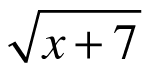= x + 1

2.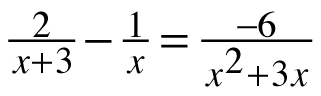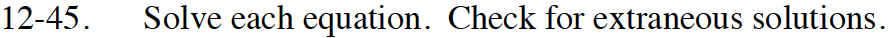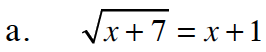Square both sides of the equation to remove the radical.

x + 7 = x2 + 2x + 1

Set the equation equal to 0 because it is quadratic (there is an x2-term.)

0 = x2 + x − 6

Factor the polynomial.

Use the Zero Product Property to solve.

Check your solutions in the original equation. Do they both work?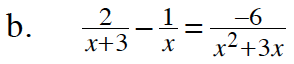Notice that you can factor x2 + 3x to x(x + 3).

Multiply both sides of the equation by a common denominator to remove the fractions.

$x(x+3)\left (\frac{2}{x+3}-\frac{1}{x} \right )\ =\ x(x+3)\left (\frac{-6}{x\left (x+3 \right )} \right )$

2x − (x + 3) = −6

Solve for x.

x = −3

Substitute your solution back into the original equation. Is x = −3 a valid solution to this problem?